# Inflationary cosmology questions

• ilikescience94
In summary, the geometry of the universe affects the rate of expansion, light paths, and overall shape.f

#### ilikescience94

Hi all, first post, just a mechanical engineer with an interest in theoretical physics. I have a few questions about inflationary cosmology that I was hoping some people could help me with. First what exactly is the bowl that inflationary cosmology talks about, I read Brian Greene's Fabric of the Cosmos, and he referred to the inflaton field falling off of its bowl, releasing energy and forming the universe as inflationary cosmology explains. What determines the shape of this bowl, and most especially, is the inflaton field at its low point, or could it be some strange bowl, with multiple peaks and trough allowing for accelerated and decelerating cycles, and possibly even contracting cycles(I drew an awful portrayal of this on paint, but it gets my idea across, is there any mathematical formula tat determines the bowls shape or is it speculative). How big is the universe, In Greene's book, he said that inflationary cosmology makes the universe so large that if scaled to the size of earth, the entire 13.7 billion light year observable universe would be a grain of sand. Is this a pretty recognized answer, is conservative, or extreme based on estimates? He also said that there was 20 pounds of matter clumped into a nugget that was something like the size of a billionth of a billionth that of an atom, more of a quantum question here, but how could matter fit together so tightly, or is that a special form of matter (perhaps dark matter or dark energy, the book was written like 6 years ago, so perhaps more theories have been formed on the subject.) Also, has the age of our universe taken cosmological inflation into account, light and more importantly Microwave Background Radiation would have to travel past the universes superluminal expansion, as well as the 7 billion years of decelerated expansion as well as the almost 7 billion years of accelerated expansion we have experienced. Also, what is the rate of accelerated expansion, I believe I heard 72km/sec/3.2million light years, is that a trusted answer?

#### Attachments

•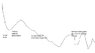Untitled.jpg
13.8 KB · Views: 394
You have numerous questions in your post, so I will cut to the chase with a couple of articles that will help narrow out your questions. One problem with Brian Greenes book is some of the analogies he uses often mislead the reader. Both articles as well as the Forum FAQ should help you catch up to current cosmology.

Universe geometry

The origins of the universe is unknown in cosmology. The hot big bang model only covers the history of the universe from 10-43 seconds forward. Prior to that is described as a singularity. However its important to note that the singularity is not a black hole style. Instead singularity in this case simply means a point in time where our mathematics can no longer accurately describe it. Numerous youtube videos and pop media articles would have you believe our universe exploded from some super particle. This was never predicted by the hot big bang model.

The observable universe which is the portion we can see is a finite, sphere with a radius of 46 Gly, which is equal to 46 billion light years. The 46 Gly particle horizon refers to the today's distance of objects, whose radiation emitted in the past we receive today. The overall size of the universe is not known, it could be infinite or finite. If its infinite now then it would be infinite in the past, a finite value can never become infinite. So why is geometry so important to cosmology if we know the size of the observable universe? The answer to that question lies in how geometry affects the following aspects, Light paths, rate of expansion or collapse and overall shape.

In regards to light paths and geometry a closed universe described as a sphere will have two beams of light emitted at different angles eventually converge. An open hyperbolic universe such as a saddlebag will have those same two light beams diverge. A flat universe will have parallel light paths (provided the beams at emission were parallel to begin with)
You will notice on each image there is a triangle, this triangle represents how the geometry affects our measurements. In a flat curvature the three angles of a equilateral triangle will add up to 1800. A positive curvature will add up to greater than 1800, a negative curvature will add up to less than 1800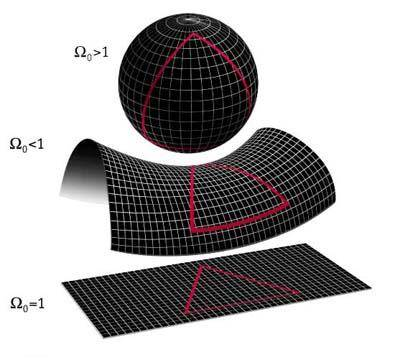The topography of the universe is determined by a comparison of the actual density (total density) as compared to the critical density. The critical density is represented by the following formula

$\rho_{crit} = \frac{3c^2H^2}{8\pi G}$

P=pressure
c=speed of light
G= gravitational constant.

density is represented by the Greek letter Omega $\Omega$ so critical density is $\Omega crit$
total density is

$\Omega$total=$\Omega$dark matter+$\Omega$baryonic+$\Omega$radiation+$\Omega$relativistic radiation+${\Omega_ \Lambda}$

$\Lambda$ or Lambda is the value of the cosmological constant often referred to as "dark energy" more accurately it is the vacuum pressure that attributes to expansion.
the subscript "0"for $\Omega$ shown in the image above denotes time in the present.

Energy-density is the amount of energy stored per unit volume of space or region. Energy per unit volume has the same physical units as pressure, and in many circumstances is an exact synonym.

$\Omega=\frac{P_{total}}{P_{crit}}$
or alternately
$\Omega=\frac{\Omega_{total}}{\Omega_{crit}}$

Geometry in 2D
In developing a theory of space-time, where curvature is related to the mass-energy density, Scientists needed a way of mathematically describing curvature. Since picturing the curvature of a four-dimensional space-time is difficult to visualize. We will start by considering ways of describing the curvature of two-dimensional spaces and progress to 4 dimensional spaces.
The simplest of two-dimensional spaces is a plane, on which Euclidean geometry holds.
This is the geometry that we learned in high school: parallel lines will go off to infinity
without ever crossing; triangles have interior angles that add up to 180. Pythagoras’
theorem which relates the lengths of the sides of a right triangle also holds:
c2 = a2 + b2
where c is the length of the hypotenuse of the right triangle, and a and b are the
lengths of the other two sides. One can generalize the Pythagorean theorem to three dimensions as well:
c2= a2 + b2 + c2
see image 2.0 below

On a plane, a "geodesic" is a straight line(shortest distance between two points). If a triangle is constructed on a flat 2 dimensional plane by connecting three points with geodesics. The curvature can be represented in 2D, if you establish each angle of a equilateral triangle with
$\alpha$,$\beta$,$\gamma$ for a flat geometry this follows the relation

$\alpha$+$\beta$+$\gamma$=$\pi$.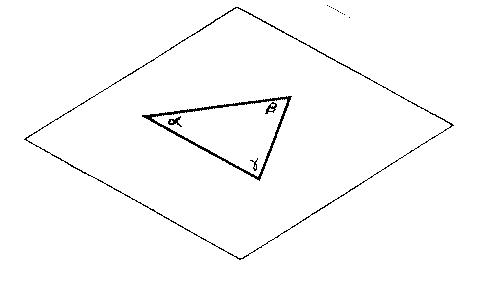image 1.0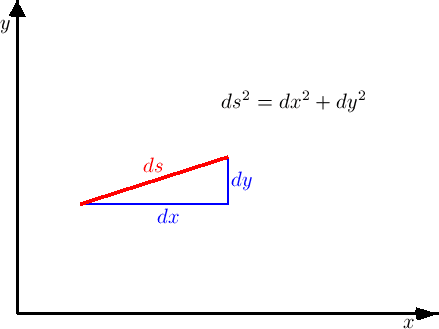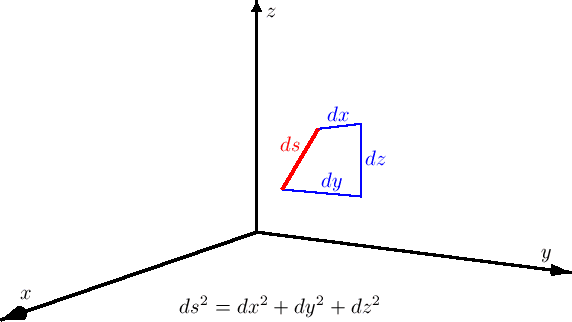On a plane, (shown above) we can set up a cartesian coordinate system, and assign to every point a coordinate (x; y). On a plane, the distance ds between points (dx and dy) is given by the relation
$d{s^2}=d{x^2}+d{y^2}$

If a triangle is constructed on the surface of the sphere by connecting the angles will obey the relation

$\alpha$+$\beta$+$\gamma$=$\pi+{AR^2}$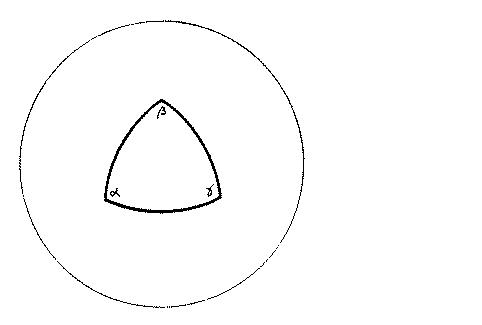image 1.1
where A is the area of the triangle, and R is the radius of the sphere. All spaces in which
$\alpha$+$\beta$+$\gamma$>$\pi$ are called positively curved" spaces. It is a space where the curvature is homogeneous and isotropic; no matter where you draw a triangle on the surface of a sphere, or how you orient it, it must always satisfy the above equation.
"On the surface of a sphere, we can set up polar coordinates "north pole" and "south pole" and by picking a geodesic from the north to south pole to be the "prime meridian". If r is the distance from the north pole, and $\theta$ is the azimuthal. angle measured relative to the prime meridian,"(1) then the distance ds between a point (r; $\theta$) and another nearby point (r+dr+$\theta$+d$\theta$) is given by the relation

${ds^2} = {dr^2} + {R^2} {sin^2}(r/R)d\theta^2$

"An example of a negatively curved two-dimensional space is the hyperboloid, or saddle-shape. A surface of constant negative curvature. The saddle-shape has constant curvature only in the central region, near the "seat" of the saddle."(1). David Hilbert proved that a constant negative curvature cannot be constructed in a Euclidean 3D space. Consider a two-dimensional surface of constant negative curvature, with radius of curvature R. If a triangle is constructed on this surface by connecting three points with geodesics, the angles at its vertices $\alpha$
$\beta$,$\gamma$ obey the relation $\alpha$+$\beta$+$\gamma$=$\pi-{AR^2}$.

${ds^2} = {dr^2} + {R^2} {sinH^2}(r/R)d\theta^2$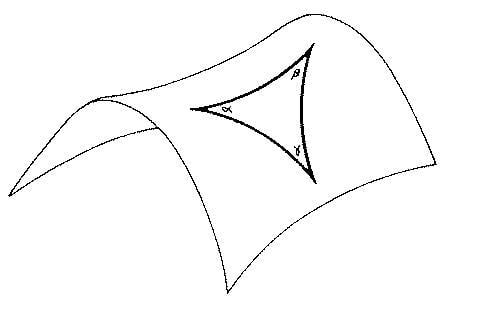image 1.2

A negative curvature is an open topography

If a two-dimensional space has curvature or flat which is homogeneous and isotropic, its geometry can
be specified by two quantities k, and R. The number k, called the curvature constant, R is the radius

k = 0 for a flat space,
k = +1 for a positively curved space,
k = -1 for a negatively curved space

Geometry in 3D
A two dimensional space can be extended to a three-dimensional space, if its curvature is homogeneous and isotropic, must be flat, or have uniform positive curvature, or have
uniform negative curvature.

The 3 possible metrics for homogeneous and isotropic 3D geometries can be represented in the form ds2=dr2=Sk(r)22

where
2=dθ2=sin2d$\phi$2

$$S\kappa(r)= \begin{cases} R sin(r/R &(k=+1)\\ r &(k=0)\\ R sin(r/R) &(k=-1) \end {cases}$$

If a three-dimensional space is flat (k = 0), it
has the metric

ds2 = dx2 + dy2 + dz2 ;

expressed in cartesian coordinates or

${ds^2} = {dr^2} +{r^2}[d\theta^2 + {sin^2} d\phi^2]$

If a three-dimensional space has uniform positive curvature (k = +1), its
metric is

${ds^2} = {dr^2} +{R^2}{sin^2}(r/R)[d\theta^2 + {sin^2}\theta d\phi^2]$

A negative curvature in the uniform portion has the metric (k=-1)

${ds^2} = {dr^2} +{R^2}{sinH^2}(r/R)[d\theta^2 + {sin^2}\theta d\phi^2]$

Geometry in 4D

Thus far we have discussed the 2 and 3 dimensional components. The Friedmann-Lemaitre-Robertson-Walker metric (FLRW) can be used to describe the 4D dimensions with the use of a(t). a(t) is the scale factor. See the redshift and expansion article for more information or the cosmocalc link on the main page. Scale factor in a homogeneous and isotropic universe describes how the universe expands or contracts with time.
The FLRW metric can be written in the form

$d{s^2}=-{c^2}d{t^2}+a({t^2})[d{r^2}+{S,k}{(r)^2}d\Omega^2]$

references
(1)"Introductory to Cosmology" Barbera Ryden"
images 1.0,1.1 and 1.2 (see (1))
(2)"Modern Cosmology" Scott Dodelson
(3)"lecture notes, Introductory to Cosmology" Dr. Ka Chan Lu

the other article. My signature has a couple of handy links with further information specifically in inflation. Also has a link to a handy tool called the lightcone calculator with which you can plot the expansion history of the universe past present and future. Feel free to ask any questions that these articles did not cover for you

Universe: A generalized definition of the universe can be described as everything that is. In Cosmology the universe can be described as everything measurable in our space-time either directly or indirectly. This definition forms the basis of the observable universe. The Hot Big Bang model does not describe prior to 10-43 seconds. The LambdaCDM or $\Lambda$CDM model is a fine tuned version of the general FLRW (Freidmann Lemaitre Robertson Walker) metrics, where the six observationally based model parameters are chosen for the best fit to our universe.

The Observable universe is 46 Billion light years, or 4.3×1026 meters with an age as of 2013, is 13.772 ± 0.059 billion years.
In the hot big bang model we do not think of the universe as starting from a singularity (infinitely, hot, dense point) instead measurements agree space-time as simply expanding. That expansion is homogeneous and isotropic. If you were to take a telescope and look at the night sky, no matter where you look the universe looks the same or homogeneous meaning no preferred location. As you change directions with the telescope you will find that no matter which direction you look the universe looks the same or isotropic meaning no preferred direction. These terms in cosmology are only accurate at certain scales. Below 100Mpc it is obvious that the universe is inhomogeneous and anisotropic. As such objects as stars and galaxies reside in this scale. This also tells us that there is no center of the universe, as a center is a preferred location. These terms also describe expansion. Expansion will be covered in more detail in the Cosmological Redshift section. Whether or not the universe is finite or infinite is not known. However if it is infinite now so it must be in the beginning.
Common misconceptions arise when one tries to visualize a finite universe such questions include.

"So how do we see farther than 13.772 billion light years?" The answer lies in expansion; as light is traveling towards us, space-time has expanded.
“If the universe is finite what exists outside the Universe?" If you think about this question with the above definition of the universe you will realize that the question is meaningless. One accurate answer in regards to cosmology is nonexistent.
"What makes up the barrier between our universe and outside our universe?" The short answer is there is no barrier.

The CMB, (Cosmic Microwave Background) The CMB is thermal radiation filling the Observable universe almost uniformly, This provides strong evidence of the homogeneous and isotropic measurements and distances. As the universe expanded, both the plasma and the radiation filling it grew cooler. When the universe cooled enough, protons and electrons combined to form neutral atoms. These atoms could no longer absorb the thermal radiation, and so the universe became transparent instead of being an opaque fog. Precise measurements of cosmic background radiation are critical to cosmology, since any proposed model of the universe must explain this radiation. CMB photons were emitted at about 3000 Kelvin and are now 2.73 Kelvin blackbody radiation. Their currently observed energy is 1/1000th of their energy as emitted.

In order to measure an objects motion and distance in cosmology it is important to properly understand redshift, Doppler shift and gravitational redshift. Incorrect usage of any of these can lead to errors in our measurements.

Doppler shift and redshift are the same phenomenon in general relativity. However you will often see Doppler factored into components with different names used, as will be explained below. In all cases of Doppler, the light emitted by one body and received by the other will be red or blueshifted i.e. its wavelength will be stretched. So the color of the light is more towards the red or blue end of the spectrum. As shown by the formula below.

$$\frac{\Delta_f}{f} = \frac{\lambda}{\lambda_o} = \frac{v}{c}=\frac{E_o}{E}=\frac{hc}{\lambda_o} \frac{\lambda}{hc}$$

The Cosmological Redshift is a redshift attributed to the expansion of space. The expansion causes a Recession Velocity for galaxies (on average) that is proportional to DISTANCE.
A key note is expansion is the same throughout the cosmos. However gravity in galaxy clusters is strong enough to prevent expansion. In other words galaxy clusters are gravitationally bound. In regards to expansion it is important to realize that galaxies are not moving from us due to inertia, rather the space between two coordinates are expanding. One way to visualize this is to use a grid where each vertical and horizontal joint is a coordinate. The space between the coordinates increase rather than the coordinates changing. This is important in that no FORCE is acting upon the galaxies to cause expansion. As expansion is homogeneous and isotropic then there is no difference in expansion at one location or another. In the $\Lambda$CDM model expansion is attributed to the cosmological constant described later on. The rate a galaxy is moving from us is referred to as recession velocity. This recession velocity then produces a Doppler (red) shift proportional to distance (please note that this recession velocity must be converted to a relative velocity along the light path before it can be used in the Doppler formula). The further away an object is the greater the amount of redshift. This is given in accordance with Hubble’s Law. In order to quantify the velocity of this galactic movement, Hubble proposed Hubble's Law of Cosmic Expansion, aka Hubble's law, an equation that states:

Hubble’s Law: The greater the distance of measurement the greater the recessive velocity

Velocity = H0 × distance.

Velocity represents the galaxy's recessive velocity; H0 is the Hubble constant, or parameter that indicates the rate at which the universe is expanding; and distance is the galaxy's distance from the one with which it's being compared.

The Hubble Constant The Hubble “constant” is a constant only in space, not in time,the subscript ‘0’ indicates the value of the Hubble constant today and the Hubble parameter is thought to be decreasing with time. The current accepted value is 70 kilometers/second per mega parsec, or Mpc. The latter being a unit of distance in intergalactic space described above.
Any measurement of redshift above the Hubble distance defined as H0 = 4300±400 Mpc will have a recessive velocity of greater than the speed of light. This does not violate GR because a recession velocity is not a relative velocity or an inertial velocity. It is precisely analogous to a separation speed. If, in one frame of reference, one object is moving east at .9c, and another west at .9c, they are separating by 1.8c. This is their recession velocity. Their relative velocity remains less than c. In cosmology, two things change from this simple picture: expansion can cause separation speeds much greater even than 2c; and relative velocity is not unique, but no matter what path it is compared along, it is always less than c, as expected.

z = (Observed wavelength - Rest wavelength)/(Rest wavelength) or more accurately

1+z= λobservedemitted or z=(λobservedemitted)/λemitted

$$1+Z=\frac{\lambda}{\lambda_o}$$ or $$1+Z=\frac{\lambda-\lambda_o}{\lambda_o}$$

λ0= rest wavelength
Note that positive values of z correspond to increased wavelengths (redshifts).
Strictly speaking, when z < 0, this quantity is called a blueshift, rather than
a redshift. However, the vast majority of galaxies have z > 0. One notable blueshift example is the Andromeda Galaxy, which is gravitationally bound and approaching the Milky Way.
WMAP nine-year results give the redshift of photon decoupling as z=1091.64 ± 0.47 So if the matter that originally emitted the oldest CMBR photons has a present distance of 46 billion light years, then at the time of decoupling when the photons were originally emitted, the distance would have been only about 42 million light-years away.

Cosmological Constant is a homogeneous energy density that causes the expansion of the universe to accelerate. Originally proposed early in the development of general relativity in order to allow a static universe solution it was subsequently abandoned when the universe was found to be expanding. Now the cosmological constant is invoked to explain the observed acceleration of the expansion of the universe. The cosmological constant is the simplest realization of dark energy, which the more generic name is given to the unknown cause of the acceleration of the universe. Indeed what we term as "Dark" energy is an unknown energy that comprises most of the energy density of our cosmos around 73%. However the amount of dark energy per m3 is quite small. Some estimates are around about 6 × 10-10 joules per cubic meter. However their is a lot of space between large scale clusters, so that small amount per m3 adds up to a significant amount of energy in total. In the De_Sitter FLRW metric (matter removed model)
this is described in the form.

Ho$\propto\sqrt\Lambda$

Another term often used for the cosmological constant is vacuum energy described originally by the false vacuum inflationary Model by A.Guth. The cosmological constant uses the symbol Λ, the Greek letter Lambda.
The dark energy density parameter is given in the form:
$\Omega_\Lambda$ which is approximately 0.685

The Doppler Redshift results from the relative motion of the light emitting object and the observer. If the source of light is moving away from you then the wavelength of the light is stretched out, i.e., the light is shifted towards the red. When the wavelength is compressed from an object moving towards you then it moves towards the blue end of the spectrum. These effects, individually called the blueshift and the redshift are together known as Doppler shifts. The shift in the wavelength is given by a simple formula

(Observed wavelength - Rest wavelength)/(Rest wavelength) = (v/c)

$$f=\frac{c+v_r}{c+v_s}f_o$$

c=velocity of waves in a medium
$$v_r$$ is the velocity measured by the source using the source’s own proper-time clock(positive if moving toward the source
$$v_s$$ is the velocity measured by the receiver using the source’s own proper-time clock(positive if moving away from the receiver)

The above are for velocities where the source is directly away or towards the observer and for low velocities less than relativistic velocities. A relativistic Doppler formula is required when velocity is comparable to the speed of light. There are different variations of the above formula for transverse Doppler shift or other angles. Doppler shift is used to describe redshift due to inertial velocity one example is a car moving away from you the light will be redshifted, as it approaches you the light and sound will be blueshifted. In general relativity and cosmology, there is a fundamental complication in this simple picture - relative velocity cannot be defined uniquely over large distances. However, it does become unique when compared along the path of light. With relative velocity compared along the path of the light, the special relativity Doppler formula describes redshift for all situations in general relativity and cosmology. It is important to realize that gravity and expansion of the universe affect light paths, and how emitter velocity information is carried along a light path; thus gravity and expansion contribute to Doppler redshift

Gravitational Redshift describes Doppler between static emitter and receiver in a gravitational field. Static observers in a gravitational field are accelerating, not inertial, in general relativity. As a result (even though they are static) they have a relative velocity in the sense described under Doppler. Because they are static, so is this relative velocity along a light path. In fact, the relative velocity for Doppler turns out to depend only on the difference in gravitational potential between their positions. Typically, we dispense with discussion of the relative velocity along a light path for static observers, and directly describe the resulting redshift as a function of potential difference. When the potential increases from emitter to receiver, you have redshift; when it decreases you have blue shift. The formula below is the gravitational redshift formula or Einstein shift off the vacuum surrounding an uncharged, non rotating, spherical mass.
$$\frac{\lambda}{\lambda_o}=\frac{1}{\sqrt{(1 - \frac{2GM}{r c^2})}}$$

G=gravitational constant
c=speed of light
M=mass of gravitational body
r= the radial coordinate (measured as the circumference, divided by 2pi, of a sphere centered around the massive body)

The rate of expansion is expressed in the $\Lambda$CDM model in terms of
The scale factor, cosmic scale factor or sometimes the Robertson-Walker scale factor parameter of the Friedmann equations represents the relative expansion of the universe. It relates the proper distance which can change over time, or the comoving distance which is the distance at a given reference in time.

d(t)=a(t)do

where d(t) is the proper distance at epoch (t)
d0 is the distance at the reference time (to)
a(t) is the comoving angular scale factor. Which is the distance coordinate for calculating proper distance between objects at the same epoch (time)
r(t) is the comoving radial scale factor. Which is distance coordinates for calculating proper distances between objects at two different epochs (time)

$$Proper distance =\frac{\stackrel{.}{a}(t)}{a}$$

The dot above a indicates change in.

the notation R(t) indicates that the scale factor is a function of time and its value changes with time. R(t)<1 is the past, R(t)=1 is the present and R(t)>1 is the future.

$$H(t)=\frac{\stackrel{.}{a}(t)}{a(t)}$$

Expansion velocity
$$v=\frac{\stackrel{.}{a}(t)}{a}$$

This shows that Hubble's constant is time dependant.

Cosmic Distance ladder, also known as Extragalactic distance scale. Is easily thought of as a series of different measurement methods for specific distance scales. Previous in the article we discussed the various forms of Redshift. These principles are used in conjunction with the following methods described below. Modern equipment now allows use spectrometry. Spectrographs of an element give off a definite spectrum of light or wavelengths. By examining changes in this spectrum and other electromagnetic frequencies with the various forms of shifts caused by relative motion, gravitational effects and expansion. We can now judge an objects luminosity where absolute luminosity is the amount of energy emitted per second.

Luminosity is often measured in flux where flux is

$$f=\frac{L}{4\pi r^2}$$

However cosmologists typically use a scale called magnitudes. The magnitude scale has been developed so that a 5 magnitude change corresponds to a differents of 100 flux.
Rather than cover a large range of those distance scales or rungs on the ladder I will cover a few of the essential steps to cosmological distance scales. The first rung on the ladder is naturally.

Direct measurements: Direct measurements form the fundamental distance scale. Units such as the distance from Earth to the sun that are used to develop a fundamental unit called astronomical unit or AU. During the orbit around the sun we can take a variety of measurements such as Doppler shifts to use as a calibration for the AU unit. This Unit is also derived by a method called Parallax.

Parallax. Parallax is essentially trigonometric measurements of a nearby object in space. When our orbit forms a right angle triangle to us and the object to be measured
With the standardized AU unit we can take two AU to form the short leg. With the Sun at a right angle to us the distance to the object to be measured is the long leg of the triangle.

Moving Cluster Parallax is a technique where the motions of individual stars in a nearby star cluster can be used to find the distance to the cluster.

Stellar parallax is the effect of parallax on distant stars . It is parallax on an interstellar scale, and allows us to set a standard for the parsec.

Standard candles A common misconception of standard candles is that only type 1A supernova are used. Indeed any known fundamental distance measurement or stellar object whose luminosity or brightness is known can be used as a standard candle. By comparing an objects luminosity to the observed brightness we can calculate the distance to an object using the inverse square law. Standard candles include any object of known luminosity, such as Cepheid’s, novae, Type 1A supernova and galaxy clusters.

My thanks to the following Contributors, for their feedback and support.

PAllen
Naty1
Jonathon Scott
marcus

Article by Mordred, PAllen[/QUOTE]

"So how do we see farther than 13.772 billion light years?" The answer lies in expansion; as light is traveling towards us, space-time has expanded.
[/QUOTE]

Thanks for taking the time to post this, this really helped a lot, only question I still have is this. If I understand this correctly is it saying that light is carried towards Earth by the expansion of space?

Thanks for taking the time to post this, this really helped a lot, only question I still have is this. If I understand this correctly is it saying that light is carried towards Earth by the expansion of space?

I'm not 100% sure what you mean, but light does not travel because of the expansion of space, it would travel regardless of any expansion. But the distances change because of expansion.

Last edited:
Thanks for taking the time to post this, this really helped a lot, only question I still have is this. If I understand this correctly is it saying that light is carried towards Earth by the expansion of space?

No, quite the opposite. Light is actually carried AWAY from us by the expansion of space. It has to fight against that expansion in order to get to us.

No, quite the opposite. Light is actually carried AWAY from us by the expansion of space. It has to fight against that expansion in order to get to us.
Then how is the observable universe 46 billion light years across if light has only had 13.7 billion years to travel?

At the time light was emitted in the early universe distances were far closer so the light you see now has already traveled part way. Keep in mind expansion varies over time, but in the local environment the rate of expansion locally is negligible to the speed of light so it will always progress towards us. At some point however we will never see light beyond that point. Assuming the rate of expansion stays constant as it is today.

Then how is the observable universe 46 billion light years across if light has only had 13.7 billion years to travel?

Light that has traveled 13.7 billion years to reach us came from an area of space that is 46 billion light years away NOW. At the time of emission that area of space was much, much closer.

Light that has traveled 13.7 billion years to reach us came from an area of space that is 46 billion light years away NOW. At the time of emission that area of space was much, much closer.

OK I get it, thanks, and how big is it estimated the whole universe is?

OK I get it, thanks, and how big is it estimated the whole universe is?

The whole universe? Do you mean observable universe + unobservable universe? Then there is no estimation. It is unknown.